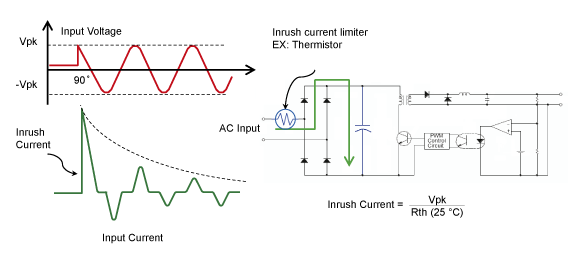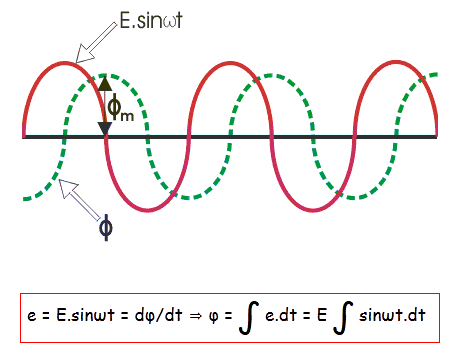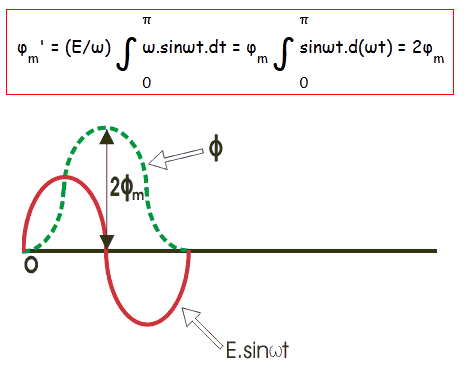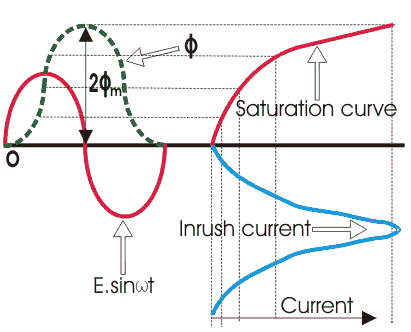# Transformer Inrush Current

Inrush Current is defined as a form of overcurrent that occurs after energizing a transformer and is a large transient current caused by part cycle saturation of the magnetic core of the transformer. For power transformers, the magnitude of inrush current is initially 6 – 10 times the rated load current.

When switching on the transformer from the primary side while its secondary circuit is kept open, it acts as a simple inductance. When the electrical power transformer works normally, the flux produced in the core is in quadrature with applied voltage.

The flux wave will reach its maximum value, 1/4 cycle or π/2 angle later, the voltage wave will reach the maximum value. According to the waves shown in the figure below, at the instant when the voltage is zero, the corresponding steady-state value of flux should be the negative maximum.

But in fact, there is impossible to have flux when we switch on the supply to the transformer. This is due to no flux linked to the core prior to switching on the supply.

The steady-state value of the flux will not be reached immediately. Although in our opinion the process is very quick - it takes a different amount of time. The rate of this process depends on how fast the circuit can take in energy.

The main reason is that the rate of energy transfer to a circuit cannot be infinity. Therefore, the flux in the core also will start from its zero value at the time of switching on the transformer. According to Faraday’s law of electromagnetic induction:

The voltage induced across the winding is calculated as: e = dφ/dt.

Where φ is the flux in the core. Hence the flux will be integral of the voltage wave, which can be calculated using the formula below:If the transformer is switched on at the instant of voltage zero, the flux wave is initiated from the same origin as voltage waveform, the value of flux at the end of the first half cycle of the voltage waveform can be calculated using:Where φm is the maximum value of the steady-state flux. The transformer core is generally saturated just above the maximum steady-state value of flux. But in our example, when switching on the transformer the maximum value of flux will jump to double its steady-state maximum value.

After the steady-state maximum value of flux, the core becomes saturated and the current required to produce the rest of the flux is very high. So, the transformer primary will draw a very high peak current from the source. This is known as the transformer inrush current or magnetizing inrush current of the transformer.Magnetizing inrush current in transformer is the current which is drown by a transformer when the transformer is energized. This current is transient in nature and exists for a few milliseconds. The inrush current may be up to 10 times higher than the normal rated current of the transformer.

In spite of the high magnitude of inrush current but there is not any permanent fault detected in the transformer due to the small time of existence.

But there is still an inrush current in the power transformer which is a problem, as it interferes with the functioning of the circuits as they were designed to work.

There are some effects of high inrush such as nuisance fuse or breaker interruptions, as well as arcing and failure of primary circuit components, such as switches. High magnetizing inrush current in transformer also require over-sizing of fuses or breakers. Another side effect of high inrush is the injection of noise and distortion back into the mains.

.(+84) 0913.006.538
Skype, Telegram, Wechat ...